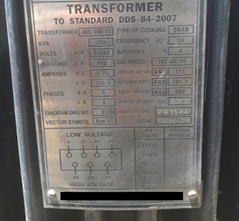# How to find the Rating of Transformer in kVA ?

In this tutorial we will see to find the Transformer Rating in KVA?
We know that, Transformers are always rated in kVA.
We can see below the two simple formulas are to find the rating of Single phase and Three phase Transformers.
P = V x I.
##### Rating of a single phase transformer in kVA
KVA= (V x I) / 1000

P = √3 V x I
##### Rating of a Three phase transformer in kVA
KVA = (√3 V x I) /1000For example the above fig. shows the rating of Transformer is 100kVA.

Primary Voltages or High Voltages (H.V) is 11000 V = 11kV.
Primary Current on High Voltage side is 5.25 Amperes.

And

Secondary voltages or Low Voltages (L.V) is 415 Volts
Secondary Current (Current on Low voltages side) is 139.1 Amp.

Now let us see how to calculate the rating of transformer according to
P=V x I (Primary voltage x primary current)
P = 11000V x 5.25A = 57,750 VA = 57.75kVA
Or P = V x I (Secondary voltages x Secondary Current)
P= 415V x 139.1A = 57,726 VA = 57.72kVA

We noticed that the rating of Transformer (on Nameplate) is 100kVA but according to calculation...it comes about 57 kVA

The difference comes due to ignorance of that we used single phase formula instead of three phase formula.

Now we will use this formula
##### P = √3 x V x I
P=√3 Vx I (Primary voltage x primary current)
P =√3 x 11000V x 5.25A = 1.732 x 11000V x 5.25A = 100,025VA (or)
P=100KVA
Or
P = √3 x V x I (Secondary voltages x Secondary Current)
P= √3 x 415V x 139.1A = 1.732 x 415V x 139.1A= 99,985 VA (or)
P= 99.98kVA

Consider the (next) following example.
Voltage (Line to line) = 208 V.
Current (Line Current) = 139 A
Now rating of the three phase transformer
##### P = √3 x V x I
P = √3 x 208 x 139A = 1.732 x 208 x 139
P = 50077 VA = 50kVA0 CommentsComments